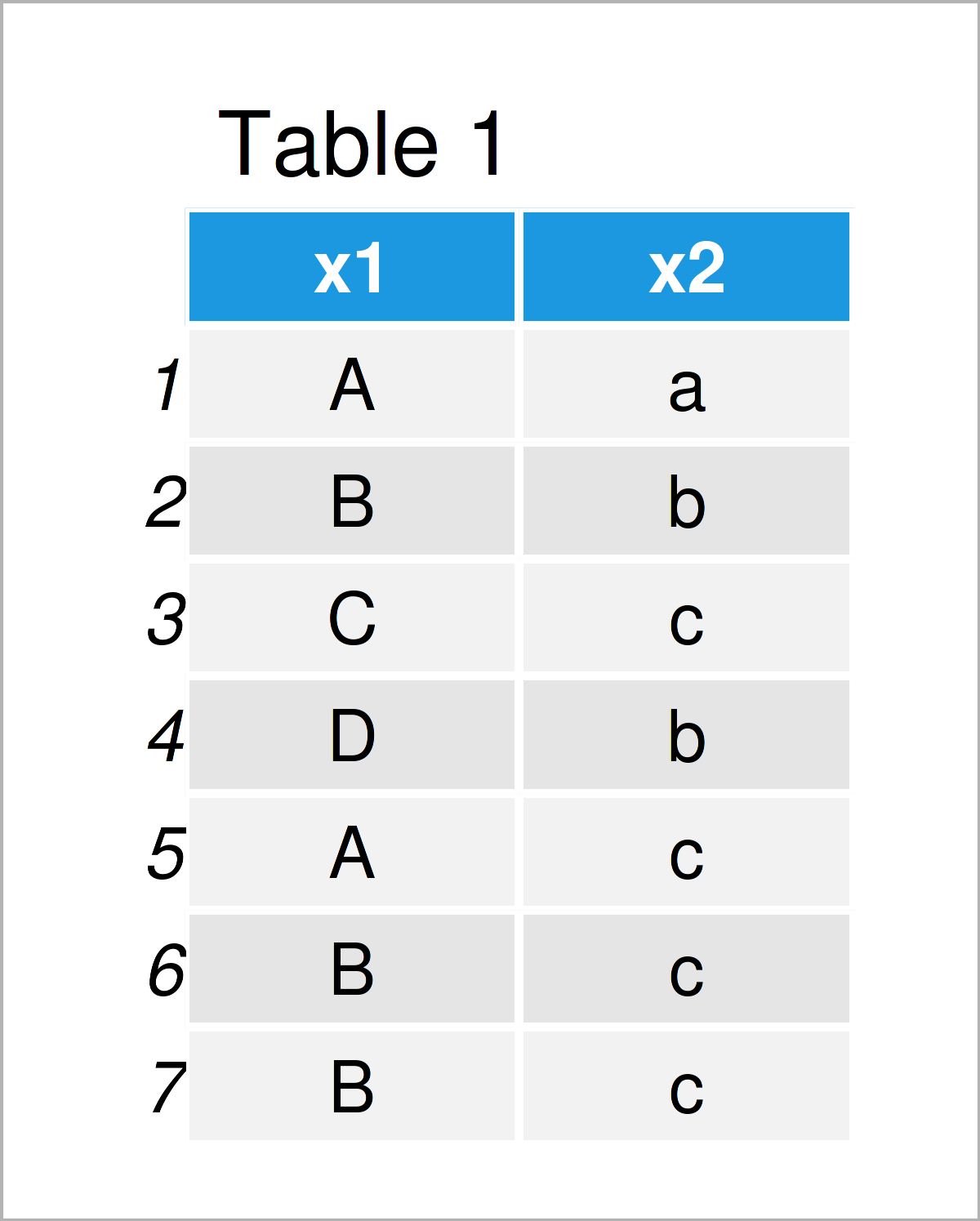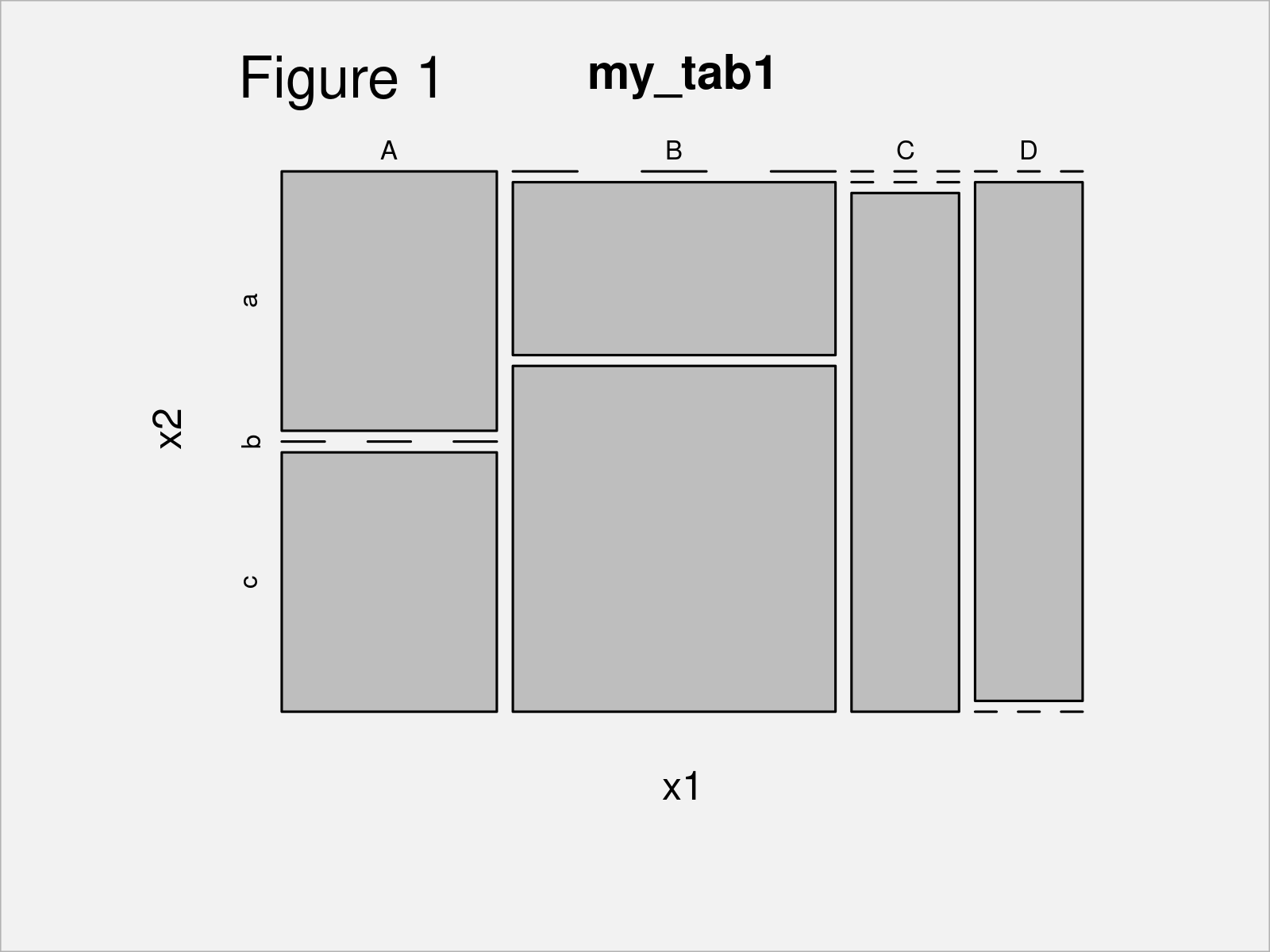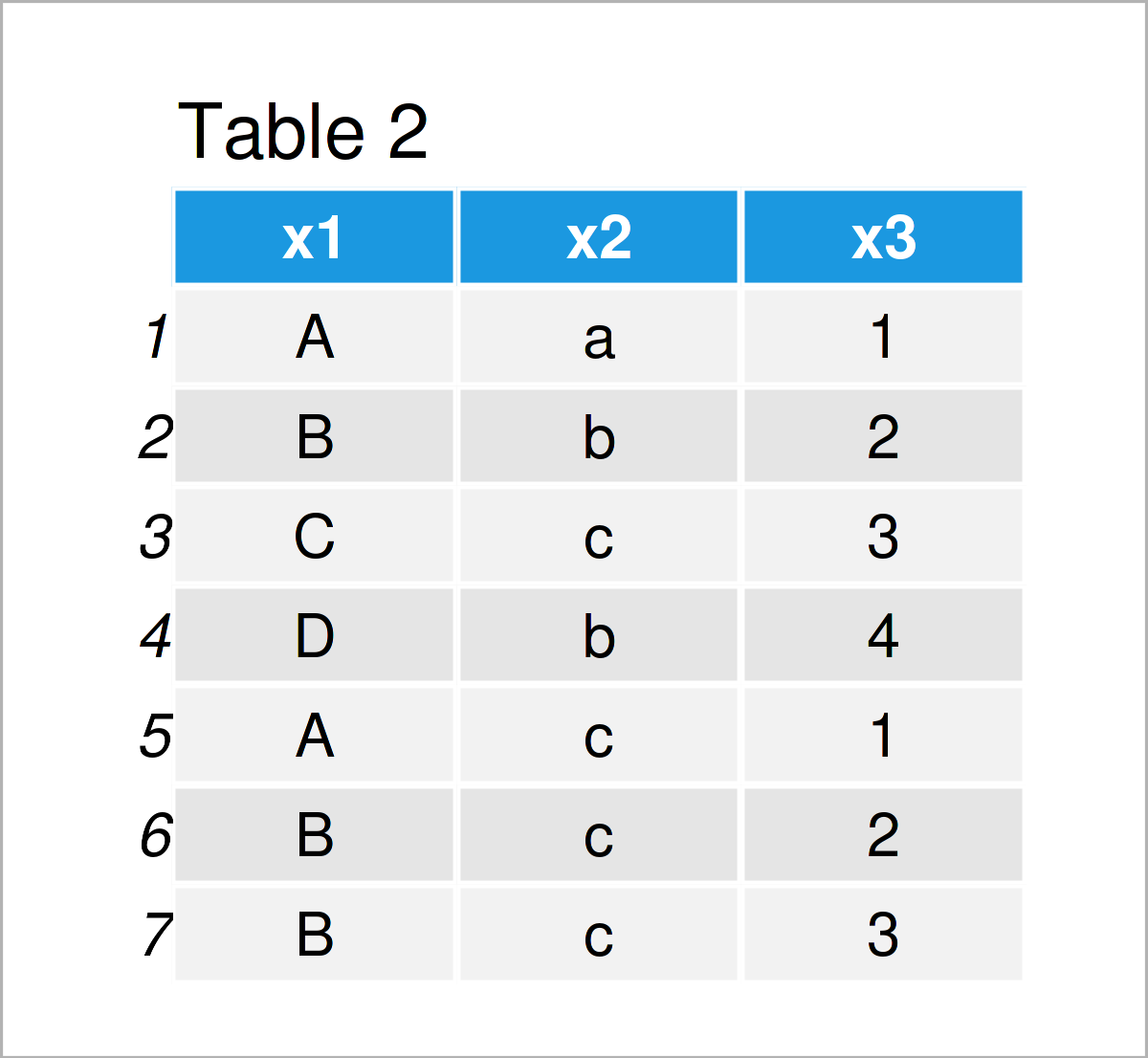# Contingency Table in R (5 Examples)

In this R tutorial you’ll learn how to make a contingency table.

The content of the article looks like this:

Here’s the step-by-step process.

## Creating Example Data

Initially, let’s construct some exemplifying data:

```data <- data.frame(x1 = c(LETTERS[1:4], "A", "B", "B"),  # Create example data frame
x2 = c(letters[1:3], "b", "c", "c", "c"))
data                                                     # Print example data frame```As you can see based on Table 1, the example data is a data frame containing seven rows and two variables.

## Example 1: Create Two-way Contingency Table

This example illustrates how to make a two-way contingency table (or cross tabulation / crosstab) in the R programming language.

For this task, we can apply the table() function to our example data frame as shown in the following R code:

```my_tab1 <- table(data)                         # Create two-way contingency table
my_tab1                                        # Print two-way contingency table
#    x2
# x1  a b c
#   A 1 0 1
#   B 0 1 2
#   C 0 0 1
#   D 0 1 0```

The previous output of the RStudio console shows a two-way cross tabulation of the two variables in our data frame. For instance, the combination of A and a occurs once, and the combination of B and a occurs not at all.

## Example 2: Draw Plot of Contingency Table

In this example, I’ll demonstrate how to draw a plot of a 2×2 contingency table.

The following R code uses the plot() function provided by Base R to create a mosaic plot of the table object we have constructed in Example 1:

`plot(my_tab1)                                  # Plot contingency table`Figure 1 shows the output of the previous R programming syntax – A mosaic graphic showing the cross tabulation of the two columns in our example data.

By the way, it is also possible to draw frequency count tables in a graph. For more info, have a look here.

## Example 3: Add Margins to Contingency Table

Example 3 shows how to add the sum of each row and each column of a table object (i.e. the margins) to this table.

To achieve this, we can apply the addmargins function as illustrated below:

```my_tab2 <- addmargins(my_tab1)                 # Add margins to contingency table
my_tab2                                        # Print contingency table with margins
#      x2
# x1    a b c Sum
#   A   1 0 1   2
#   B   0 1 2   3
#   C   0 0 1   1
#   D   0 1 0   1
#   Sum 1 2 4   7```

The previous table contains the same values as the table we have created in Example 1, plus the margins of each row and column.

## Example 4: Create Contingency Table with Proportions

The following R programming syntax shows how to get a two-way contingency table with proportions (or probabilities).

To do this, we can apply the prop.table function as shown in the following R syntax:

```my_tab3 <- prop.table(my_tab1)                 # Contingency table with proportions
my_tab3                                        # Print contingency table with proportions
#    x2
# x1          a         b         c
#   A 0.1428571 0.0000000 0.1428571
#   B 0.0000000 0.1428571 0.2857143
#   C 0.0000000 0.0000000 0.1428571
#   D 0.0000000 0.1428571 0.0000000```

## Example 5: Create Three-way Contingency Table

So far, we have created different types of two-way contingency tables. However, it is also possible to perform a three-way cross tabulation.

For this, we first have to extend our example data frame by another variable:

```data_three <- data.frame(data,                 # Create data frame with three variables
x3 = c(1:4, 1:3))
data_three                                     # Print data frame with three variables```Table 2 shows the output of the previous R programming code – i.e. a data frame with three variables.

Next, we can apply the table command to this data frame to create a three-way contingency table:

```my_tab4 <- table(data_three)                   # Create three-way contingency table
my_tab4                                        # Print three-way contingency table
# , , x3 = 1
#
#    x2
# x1  a b c
#   A 1 0 1
#   B 0 0 0
#   C 0 0 0
#   D 0 0 0
#
# , , x3 = 2
#
#    x2
# x1  a b c
#   A 0 0 0
#   B 0 1 1
#   C 0 0 0
#   D 0 0 0
#
# , , x3 = 3
#
#    x2
# x1  a b c
#   A 0 0 0
#   B 0 0 1
#   C 0 0 1
#   D 0 0 0
#
# , , x3 = 4
#
#    x2
# x1  a b c
#   A 0 0 0
#   B 0 0 0
#   C 0 0 0
#   D 0 1 0```

As you can see, the previous output has created a list object. Each element of this list shows a two-way contingency table given a certain value in the third variable of our input data.

For instance, the first list element of the previously shown list contains a two-way contingency table for the case when x3 is equal to the value 1.

## Video & Further Resources

Have a look at the following video on the Statistics Globe YouTube channel. I explain the R programming codes of this article in the video.

In addition, you may want to have a look at some of the other tutorials on this website. Some articles can be found below:

You have learned in this tutorial how to calculate and create a contingency table in R. Don’t hesitate to let me know in the comments, if you have any further questions.

Subscribe to the Statistics Globe Newsletter

•Salwa Mousa
September 11, 2022 3:03 pm

Please discuss the topic about generate And simulation from CDF not close form and How to use the panelize maximum likelihood estimation in R code

•September 19, 2022 11:10 am

Hi Salwa,

Thank you for the topic suggestion! I’ll keep this in mind for future tutorials.

Regards,
Joachim

•Neena
October 10, 2022 8:13 pm

Hi Joachim.

Great post. It was really informative.

Could you please demonstrate how one would go about returning joint and conditional probabilities from a contingency table using R?

Regards
Neena

•November 14, 2022 11:58 am

Hey Neena,

Thank you for the kind comment, glad you like the tutorial!

Please excuse the late response. I was on a long holiday so unfortunately I wasn’t able to reply sooner. Still need help with your code?

Regards,
Joachim

•Neena
November 14, 2022 4:12 pm

Hi Joachim,

Thank you for your response. I have managed to get the code.
Thank you once again.

Regards
Neena

•November 15, 2022 8:25 am